# Lattice Effect

When I initially viewed the 256-byte intro Puls by Řrřola that took first place at Riverwash 2009, I think I shared a common sentiment with many other developers out there: Thats impossible! Řrřola was kind enough to release his x86 assembly language source code, revealing that the effect is computed without the aid of 3D libraries or hardware acceleration. Its mind-blowing.

Check out the start of the source:

```  org 100h ; assume ah=bx=0 cx=255 sp=di=-2 si=100h

mov  al,13h   ;<(byte)[100h]>>8 = 0.6875
push bx       ; (word)[100h]>>16 = 0.0769
mov  dx,3C8h  ; (float)[100h] = -0.0008052
int  10h
```

The interrupt call changes the video mode to 13h (320×200 resolution with 256 colors). 3c8h is one of the ports involves in changing the palette, which is used later.

Simple enough so farno wait! What are those comments to the right? To understand them, I fired up debug:

```C:\>debug
-a
13E7:0100 mov al, 13
13E7:0102 push bx
13E7:0103 mov dx, 3c8
13E7:0106 int 10
13E7:0108
-d
13E7:0100  B0 13 53 BA C8 03 CD 10-00 00 00 00 00 00 00 00   ..S.............
13E7:0110  00 00 00 00 00 00 00 00-00 00 00 00 34 00 D6 13   ............4...
13E7:0120  00 00 00 00 00 00 00 00-00 00 00 00 00 00 00 00   ................
13E7:0130  00 00 00 00 00 00 00 00-00 00 00 00 00 00 00 00   ................
13E7:0140  00 00 00 00 00 00 00 00-00 00 00 00 00 00 00 00   ................
13E7:0150  00 00 00 00 00 00 00 00-00 00 00 00 00 00 00 00   ................
13E7:0160  00 00 00 00 00 00 00 00-00 00 00 00 00 00 00 00   ................
13E7:0170  00 00 00 00 00 00 00 00-00 00 00 00 00 00 00 00   ................
-
```

The important part is those first 4 bytes: `B0 13 53 BA`. Based on the comments, I punched those values into some test Java code:

 ```     System.out.format("%f%n", 0xB0 / 256.0);     System.out.format("%f%n", 0x13B0 / (256.0 * 256.0));     System.out.format("%f%n", Float.intBitsToFloat(0xBA5313B0));```

Which outputs:

```0.687500
0.076904
-0.000805```

Push BX maps to 53. The calls only purpose appears to be to contribute to these constants. This kind of trick reminds me of how some biological viruses compress their genome by overlapping genes (e.g. HepatitisB virus (HBV) genome). This truly is sick coding.

The compression tricks certainly dont end there, but I really wasnt that interested in how Řrřola packed the effect into 256 bytes. I just wanted to understand the mathematics behind it and what algorithm enabled it to animate in real-time. I wanted to know how fast something like it could run in Java. According to Řrřolas web site, he originally developed the concept in C and he later crunched it down to assembly, and in so doing, he obfuscated the details. At the time of this writing, Řrřola has yet to release the C code. If and when he does, Ill probably attempt a direct Java port. Until then, I decided to explore a similar lattice effect.

The code is obfuscated, but well commented. A cursory analysis of the comments suggests that ray casting is used or more specifically ray marching using some kind of distance field to speed it up. Since I wasnt inhibited by the 256-byte size constraint, I decided to go for something that may run a tad faster. I created a ray caster that intersects spheres and cylinders.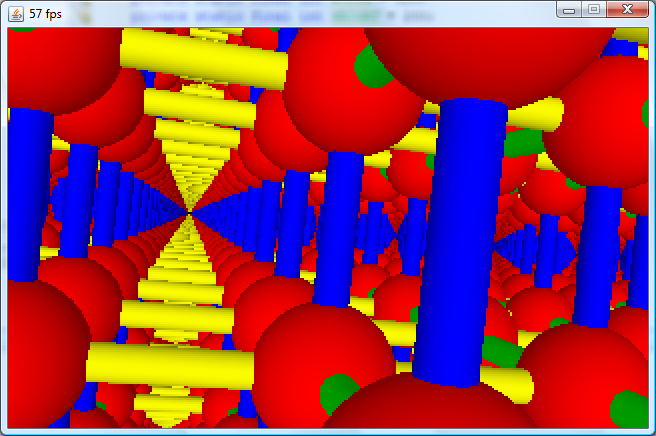Click here to launch lattice effect via Java Web Start (pinhole camera Version).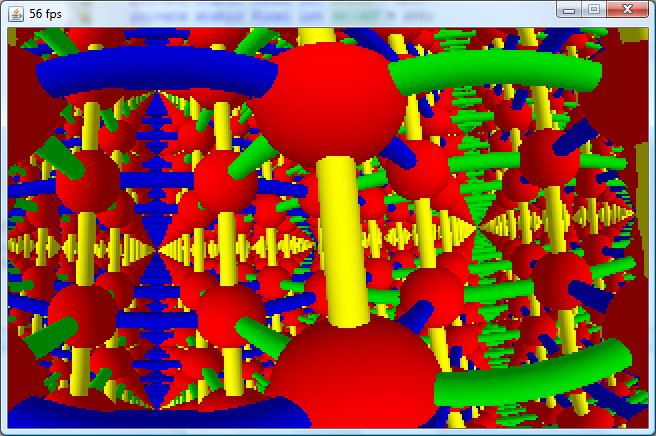Click here to launch lattice effect via Java Web Start (fisheye lens version).

I did not include any timing code. If you launch it, it will push one of your cores to the max. The frame rates above were achieved on a 2.67 GHz i7 CPU.

 ```     int[] pixels = new int[PIXELS];     float[][] rays = new float[PIXELS];     if (FISH_EYE_LENS) {       float MAX = Math.max(WIDTH, HEIGHT);       float X_OFFSET = WIDTH < HEIGHT ? (HEIGHT - WIDTH) / 2 : 0;       float Y_OFFSET = HEIGHT < WIDTH ? (WIDTH - HEIGHT) / 2 : 0;       for(int y = 0, k = 0; y < HEIGHT; y++) {         for(int x = 0; x < WIDTH; x++, k++) {           float theta = (float)(Math.PI * (0.5f + y + Y_OFFSET) / (float)MAX);           float phi = (float)(Math.PI * (0.5f + x + X_OFFSET) / (float)MAX);           float rx = (float)(Math.cos(phi) * Math.sin(theta));           float ry = (float)(Math.sin(phi) * Math.sin(theta));           float rz = (float)(Math.cos(theta));           float[] ray = rays[k];           ray = rx;           ray = ry;           ray = rz;         }       }     } else {       for(int y = 0, k = 0; y < HEIGHT; y++) {         for(int x = 0; x < WIDTH; x++, k++) {           float X = x - HALF_WIDTH + 0.5f;           float Y = -(y - HALF_HEIGHT + 0.5f);           float[] ray = rays[k];           float rx = X;           float ry = Y;           float rz = -Z0;           float inverseMag = 1f / (float)Math.sqrt(rx * rx + ry * ry + rz * rz);           ray = rx * inverseMag;           ray = ry * inverseMag;           ray = rz * inverseMag;         }       }     }```

The code starts out by pre-computing normalized ray vectors for every pixel on the screen. The fisheye lens version scans the surface of a hemisphere using the spherical coordinates formulas. The pinhole camera version computes rays from a point observer sitting a distance Z0 away from the center of a virtual screen.

 ```     while(true) {       float cosPhi = (float)Math.cos(phi);       float sinPhi = (float)Math.sin(phi);       float cosTheta = (float)Math.cos(theta);       float sinTheta = (float)Math.sin(theta);       float ux = cosPhi * sinTheta;       float uy = sinPhi * sinTheta;       float uz = cosTheta;       float vx = cosPhi * cosTheta;       float vy = sinPhi * cosTheta;       float vz = -sinTheta;       float wx = uy * vz - uz * vy;       float wy = uz * vx - ux * vz;       float wz = ux * vy - uy * vx;```

The main part of the code is an infinite loop. At the top of the loop, I apply the spherical coordinates formulas again to establish an orthonormal basis for modifying the virtual camera direction. Using 2 angles for a virtual camera is less than desirable, but all I need to do for this application is spin the camera.

The application renders an infinite lattice consisting of cube-shaped cells. At the center of each cell is a red sphere. Three cylinders pierce the sphere in the direction of each axis. Below is a rendering of only a few cells close to the camera, revealing how each cell contains three cylinders and a sphere.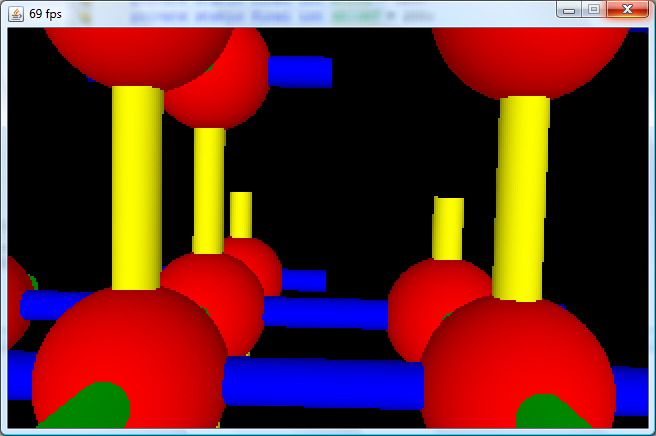The program borrows an optimization technique from ray tracing called regular grids. An excellent discussion of regular grids can be found in Ray Tracing from the Ground Up, but Ill briefly discuss it here. Rays are cast from the virtual camera out into the scene. The algorithm performs ray intersection tests for all of the objects in the cell containing the virtual camera. If the ray fails to hit anything, it advances to one of the neighboring cell and so on. In this way, a minimal number of cells need to be processed.

In the code below, the location of the observer is converted into grid cell coordinates:

 ```       int GX = (int)Math.floor(ox * INVERSE_GRID_SIZE);       int GY = (int)Math.floor(oy * INVERSE_GRID_SIZE);       int GZ = (int)Math.floor(oz * INVERSE_GRID_SIZE);```

In case you are wondering why I bothered to use the floor function, this should clear it up:

 ```     System.out.format("%d %d%n",         (int)(123 / GRID_SIZE), (int)Math.floor(123 / GRID_SIZE));     System.out.format("%d %d%n",         (int)(-123 / GRID_SIZE), (int)Math.floor(-123 / GRID_SIZE));```

Which outputs:

```0 0
0 -1```

Next, the orthnormal basis is applied to one of the pre-calculated rays (i.e. the pre-calculated ray is rotated appropriately as the camera direction demands).

 ```         float rx = ux * Rx + vx * Ry + wx * Rz;         float ry = uy * Rx + vy * Ry + wy * Rz;         float rz = uz * Rx + vz * Ry + wz * Rz;```

The regular grid algorithm advances between cells similar to Bresenham's line algorithm. It considers the evenly-spaced, infinite planes that separates cells. You can think of a ray like a particle traveling at constant rate away from the observer. The components of the particles velocity vector are independent of each other. Meaning, the time required for the particle to traverse the space between two neighboring, parallel planes is always constant. There is a constant time for each axial direction. The algorithm starts out by computing the times in each axial direction required to intersect the nearest infinite plane. Then it successively adds to those times the constant times required to cross the boundary between neighboring parallel planes. To advance the particle in the right order, it always compares all 3 times, uses the smallest and then only adjusts that time.

Below, the code uses the direction of the ray to find the times to the nearest infinite planes. It also stores the independent cell advancement direction steps (+1 or -1).

 ```         if (rx > 0) {           dgx = 1;           tx = ((GRID_SIZE * (gx + 1)) - ox) * irx;         } else {           dgx = -1;           tx = ((GRID_SIZE * gx) - ox) * irx;         }         if (ry > 0) {           dgy = 1;           ty = ((GRID_SIZE * (gy + 1)) - oy) * iry;         } else {           dgy = -1;           ty = (GRID_SIZE * gy - oy) * iry;         }         if (rz > 0) {           dgz = 1;           tz = ((GRID_SIZE * (gz + 1)) - oz) * irz;         } else {           dgz = -1;           tz = ((GRID_SIZE * gz) - oz) * irz;         }```

The time required to traverse the space between two neighboring, parallel planes is computing by using the distance between the planes.

 ```         float dtx = Math.abs(GRID_SIZE * irx);         float dty = Math.abs(GRID_SIZE * iry);         float dtz = Math.abs(GRID_SIZE * irz);```

At the bottom of the loop, it figures out which of the 3 times is the smallest. It advances the cell coordinates in the associated direction and adds the constant intra-plane time to the time.

 ```           if (tx < ty) {             if (tx < tz) {               tx += dtx;               gx += dgx;             } else {               tz += dtz;               gz += dgz;             }           } else if (ty < tz) {             ty += dty;             gy += dgy;           } else {             tz += dtz;             gz += dgz;           }```

An inner-loop steps through the grid using this technique up to a specified number of iterations. During each iteration, it performs the four intersection tests. First, it tries to hit each cylinder and then it tests the sphere. Detecting a hit is not sufficient; all four intersection tests must be performed to find the object closest to the observer. A discussion of the math behind the intersection tests can be found here. Most of the code is redundant in an attempt to speed it up.

Coloring the objects is based on the Phong shading model. Ambient and diffuse shading is used. The light direction is parallel to one of the axes of the orthonormal basis, moving with the camera and providing a consistent shading.

I cant determine if the regular grid optimization is used in the Puls intro, but the comments reveal a lot. Here are some key comments:

```; "ambient occlusion" strength (default 86: -1 byte)

;fisheye projection: z = C-x*x-y*y

;intersect ray with scene, count number of bounding volume misses

;raycasting using unbounded binary search

;last probe was inside: halve step and go back
;              outside: double step and go forward

;bounding volumes: "blow up" the scene by the current step size

;green octahedra:              (|x|+|y|+|z|)/2 - 0.1445 + r < blowup
;orange octahedra: (|x+0.5|+|y+0.5|+|z+0.5|)/2 - 0.1445 - r < blowup

;bars:  (||x|-|z|| + ||y|-|x|| + ||z|-|y||)/2 - 0.0676 < blowup
;bolts: (||x|-|z|| + ||y|-|x|| + ||z|-|y||)/2 - 0.1445 < blowup

;adjust step size according to the hit result
```

I like how ambient occlusion is in quotes. Real ambient occlusion is a computationally expensive technique used in ray tracing. It casts secondary rays out from the surfaces of objects to estimate how much open space there is between the object and its neighbors. If there is not a lot of open space, then not that much light can enter and the surface is shaded darker. I know there are tricks to approximate something like it in real-time, but the quotes hint that it is faked.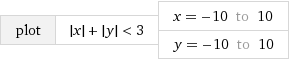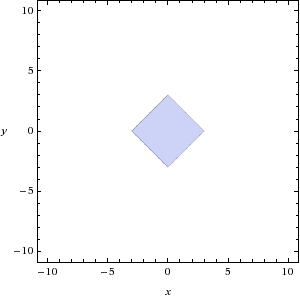As the comment suggests, in 3D, |x| + |y| + |z| < R confines an octahedron with bounds of ±R in each axis. This was very surprising. I originally thought that the image was a distorted view of a simple cubic lattice. The vertices in such a lattice would be connected to 6 edges, each parallel to an axis. And, since the struts representing the edges connect perpendicularly to the faces of the volume representing the vertex, each vertex volume would be a cube. But, the vertex volumes are actually octahedrons, meaning 8 edges connect to each vertex. In 2D, only 4 edges: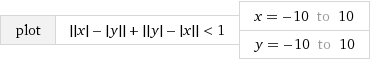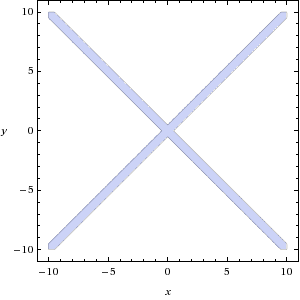To visualize an octahedral lattice, check out the structure of cesium chloride:The structure is known as a body-centered cubic system because it has one vertex in the center of each cubic cell in addition to the corner vertices. Each corner vertex is shared by 8 cubic cells. Hence, each corner vertex has edges to 8 body-centered vertices. If you remove the horizontal and vertical edges, what remains is an octahedral lattice. Click here for a second visualization.

The 0.5 offset in the inequality for the orange octahedron suggests that lattice consists of repeated unit cubes.

The size of the octahedrons and the thickness of the bars are easy to control by changing the values on the right-side of the inequalities. The moving nuts (Řrřola inadvertently called them bolts) are a little more complicated. A nut is essentially a thicker bar bound between 2 octahedrons. As the sizes of those 2 octahedrons change together, the nut appears to slide on the bar. Below, you can see 4 nuts in the 2D versions of the inequalities. The nuts consists of bars of size 3 trapped between octahedrons of sizes 4 and 6.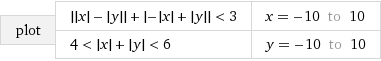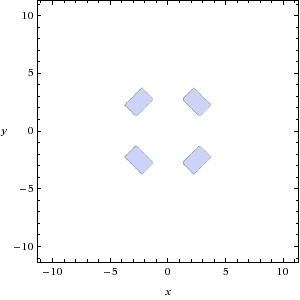To render these shapes in 3D, I modified the inner-loop of my program a bit. It still uses the regular grid optimization, but instead of intersecting spheres and cylinders, it performs ray marching. The algorithm is based on my interpretation of Řrřolas comments. The inequalities above, can be written as:

f(x, y, z) < R

As you change R, the size of the bounded volume changes in the direction of the surface gradient. For example, to move a point on the surface of the volume by a distance equal to the magnitude of the gradient at that point, you can do this:

f(x, y, z) < R + 1

Or, if you know the magnitude of the gradient at that point, call it M, then you can a move point on the surface by some distance S:

f(x, y, z) < R + S/M

The algorithm works by setting S to a small value and then it iteratively doubles S until the location of the (stationary) marching particle satisfies the inequality. Once it does, it halves S. Now, there is a distance of at least S between the particle and a point on the surface. The particle makes a step along the ray by that distance and then the process is repeated, starting again with the smallest value of S. It stops when the inequality is satisfied for S below some threshold.

Oddly, shading is a function of the total number of doubling-S iterations required before the marching particle intersects an object. That is the ambient occlusion.

Unfortunately, when I plug all that in, the frame-rate is dismal. It can probably run excellently in Java if I reduce those shapes to triangles and perform ray-triangle intersection tests instead.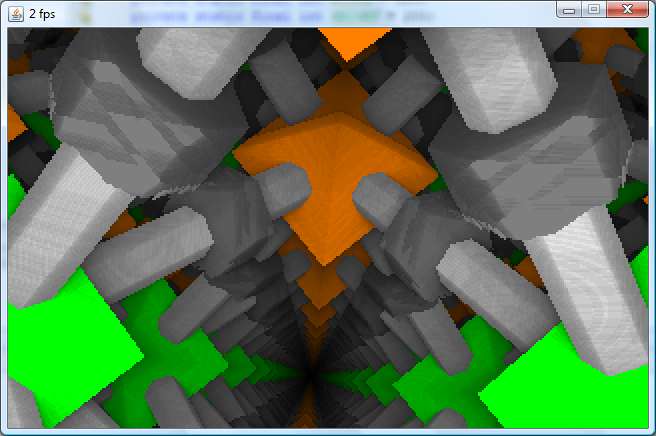Click here to launch lattice effect via Java Web Start (pinhole camera Version).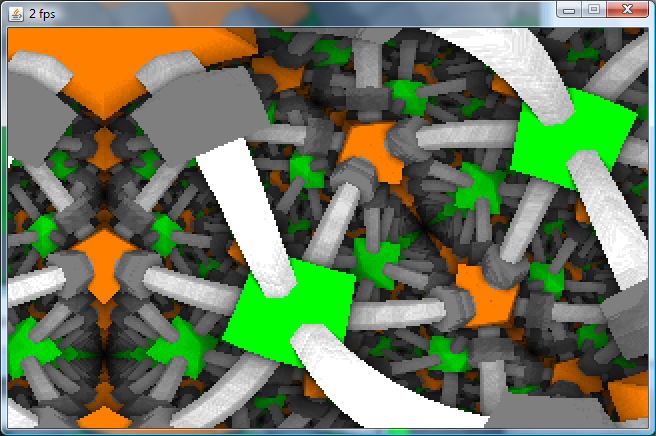Click here to launch lattice effect via Java Web Start (fisheye lens version).

### 2009.09.27

Let us all eat lettuce on the lattice.